# TypeError: can't compare datetime.datetime to datetime.dateLast updated: Jun 29, 2023
4 min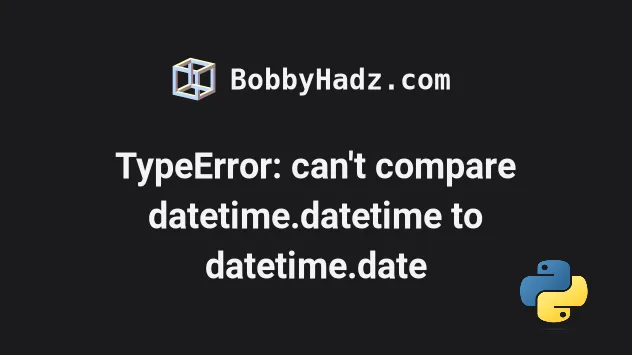## #TypeError: can't compare datetime.datetime to datetime.date

The Python error "TypeError: can't compare datetime.datetime to datetime.date" occurs when you try to compare a `datetime` object with a date.

To solve the error, use the `datetime.date()` method to convert the `datetime` object to a date before the comparison.

Here is an example of how the error occurs.

main.py
```Copied!```from datetime import datetime, date

dt = datetime(2024, 3, 15, 12, 0, 0)

print(dt)  # 👉️ 2024-03-15 12:00:00

d = date(2024, 2, 10)
print(d)  # 👉️ 2024-02-10

# ⛔️ TypeError: can't compare datetime.datetime to datetime.date
if d < dt:
print('d is less than dt')
elif d > dt:
print('d is greater than dt')
else:
print('d is equal to dt')
``````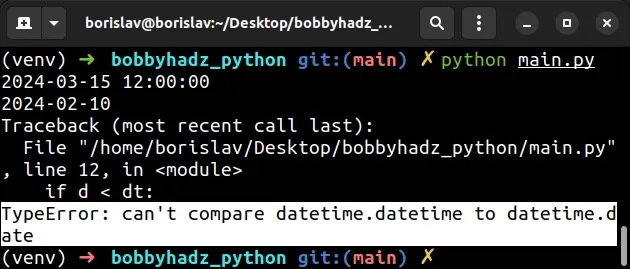We used the datetime.datetime class to create a `datetime` object.

Notice that the object contains the date and the time.

We then used the datetime.date class to create a `date` object.

Notice that the `date` object only contains the date.

You might have also used the datetime.now method to create a `datetime` object.

main.py
```Copied!```from datetime import datetime, date

# 👇️ 2023-06-29 06:45:10.137118
print(datetime.now())
``````

## #Convert the `datetime` object to a `date` to solve the error

One way to solve the error is to convert the `datetime` object to a `date`.

You can use the datetime.date method to return a `date` object with the same year, month and day as the `datetime` object.

main.py
```Copied!```from datetime import datetime, date

dt = datetime(2024, 3, 15, 12, 0, 0)

print(dt)  # 👉️ 2024-03-15 12:00:00

d = date(2024, 2, 10)
print(d)  # 👉️ 2024-02-10

# ✅ Convert to date object
if d < datetime.date(dt):
print('d is less than dt')
elif d > dt:
print('d is greater than dt')
else:
print('d is equal to dt')
``````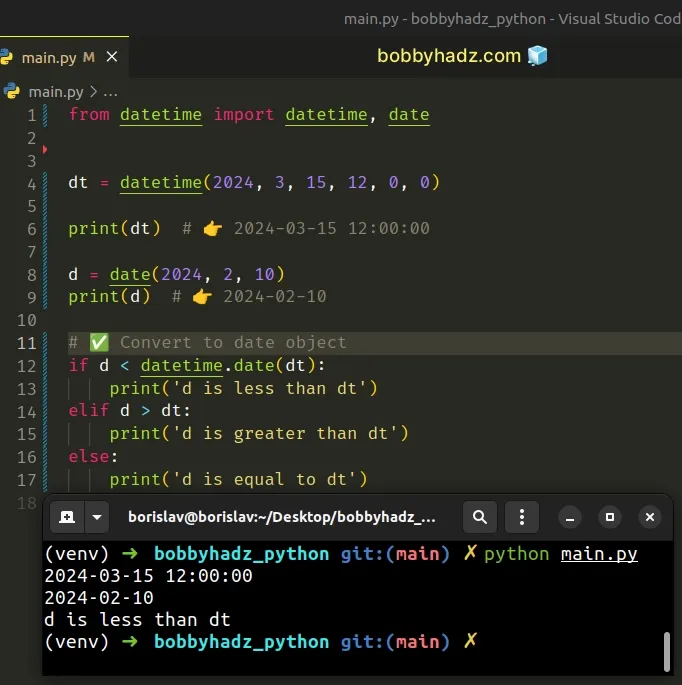Notice that I passed the `datetime` object to the `datetime.date()` class to convert it to a `date` object when comparing.

main.py
```Copied!```if d < datetime.date(dt):
``````

We could've also converted the `datetime` object to a `date` object directly upon creation.

main.py
```Copied!```from datetime import datetime, date

# ✅ Convert datetime object to date object
dt = datetime.date(datetime(2024, 3, 15, 12, 0, 0))
print(dt)  # 👉️ 2024-03-15

d = date(2024, 2, 10)
print(d)  # 👉️ 2024-02-10

if d < dt:
print('d is less than dt')
elif d > dt:
print('d is greater than dt')
else:
print('d is equal to dt')
``````

After converting the `datetime` object to a `date` object, we have 2 `date` objects, so the comparison is allowed.

## #Using the `datetime.combine()` method to solve the error

Alternatively, you can solve the error by converting the `date` object to a `datetime` object and comparing the two `datetime` objects.

main.py
```Copied!```from datetime import datetime, date, time

dt = datetime(2024, 3, 15, 12, 0, 0)
print(dt)  # 👉️ 2024-03-15 12:00:00

# ✅ Convert to datetime object
d = datetime.combine(date(2024, 2, 10), time(0, 0))
print(d)  # 👉️ 2024-02-10 00:00:00

if d < dt:
print('d is less than dt')
elif d > dt:
print('d is greater than dt')
else:
print('d is equal to dt')
``````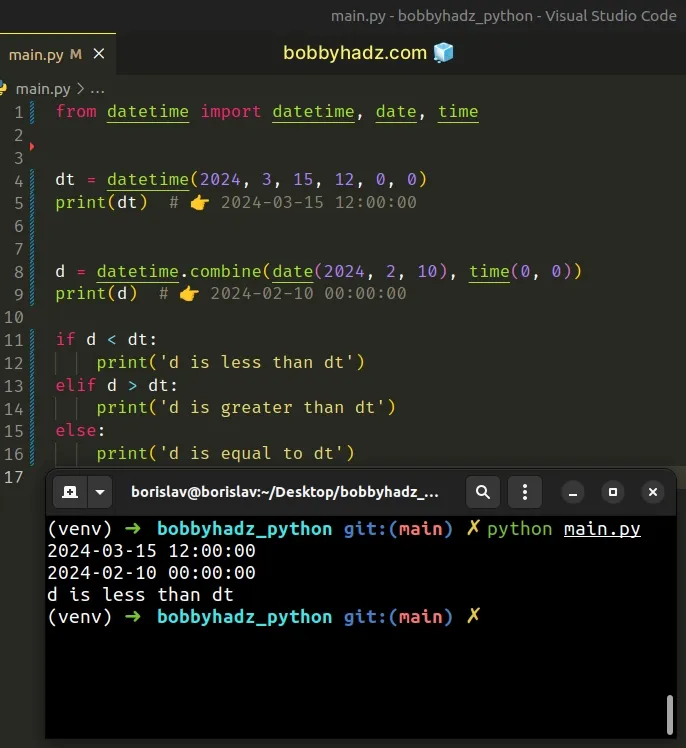The datetime.combine method returns a new `datetime` object whose date components are equal to the given date object's components and whose time components are equal to the given time object's components.

Once we convert the `date` object to a `datetime` object, we can compare the two datetime objects without any issues.

You can also convert the `date` object to a `datetime` object when comparing instead of upon instantiation.

main.py
```Copied!```from datetime import datetime, date, time

dt = datetime(2024, 3, 15, 12, 0, 0)
print(dt)  # 👉️ 2024-03-15 12:00:00

d = date(2024, 2, 10)
print(d)  # 👉️ 2024-02-10

# ✅ Convert date object to datetime object here
if datetime.combine(d, time(0, 0)) < dt:
print('d is less than dt')
elif d > dt:
print('d is greater than dt')
else:
print('d is equal to dt')
``````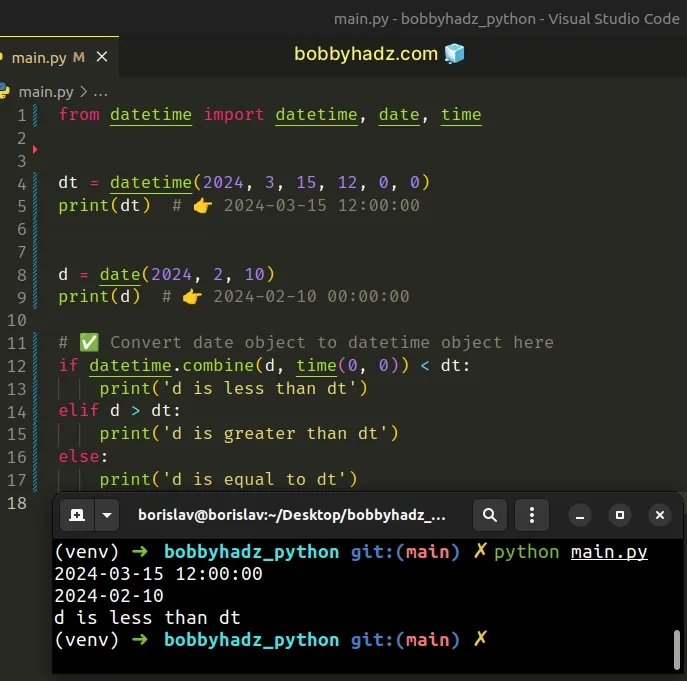The first argument the `datetime.combine()` method takes is a `date` object and the second is a `time` object.

The method combines the `date` and `time` objects to produce a `datetime` object.

## #Using the `date()` attribute to solve the error

You can also solve the error by calling the `date()` method on the `datetime` object.

main.py
```Copied!```from datetime import datetime, date

dt = datetime(2024, 3, 15, 12, 0, 0)
print(dt)  # 👉️ 2024-03-15 12:00:00

d = date(2024, 2, 10)
print(d)  # 👉️ 2024-02-10

# ✅ Call date() on datetime object
if d < dt.date():
print('d is less than dt')
elif d > dt:
print('d is greater than dt')
else:
print('d is equal to dt')
``````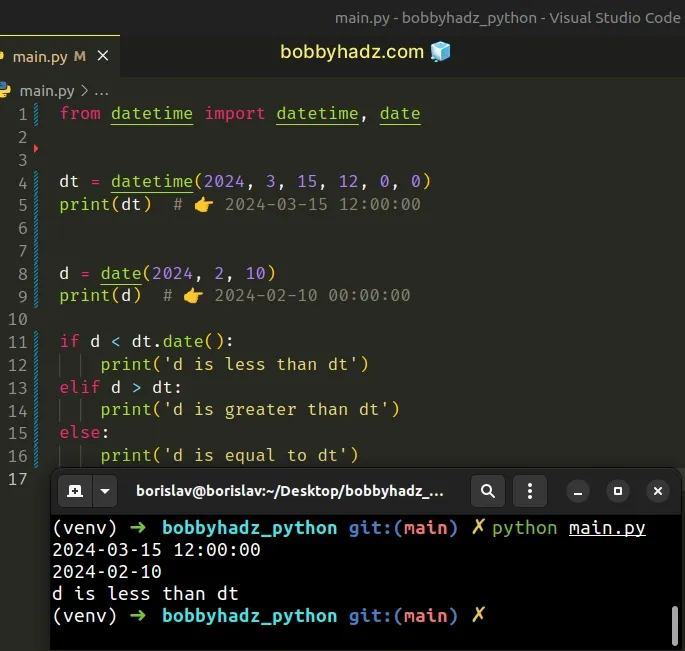We called the `date()` method on the `datetime` object to convert the `datetime` object to a `date` object.

Comparing the two `date` objects is allowed, so the error is resolved.

You can also call the `date()` method on the `datetime` object upon instantiation.

main.py
```Copied!```from datetime import datetime, date

# ✅ calling date() method here instead
dt = datetime(2024, 3, 15, 12, 0, 0).date()
print(dt)  # 👉️ 2024-03-15

d = date(2024, 2, 10)
print(d)  # 👉️ 2024-02-10

if d < dt:
print('d is less than dt')
elif d > dt:
print('d is greater than dt')
else:
print('d is equal to dt')
``````